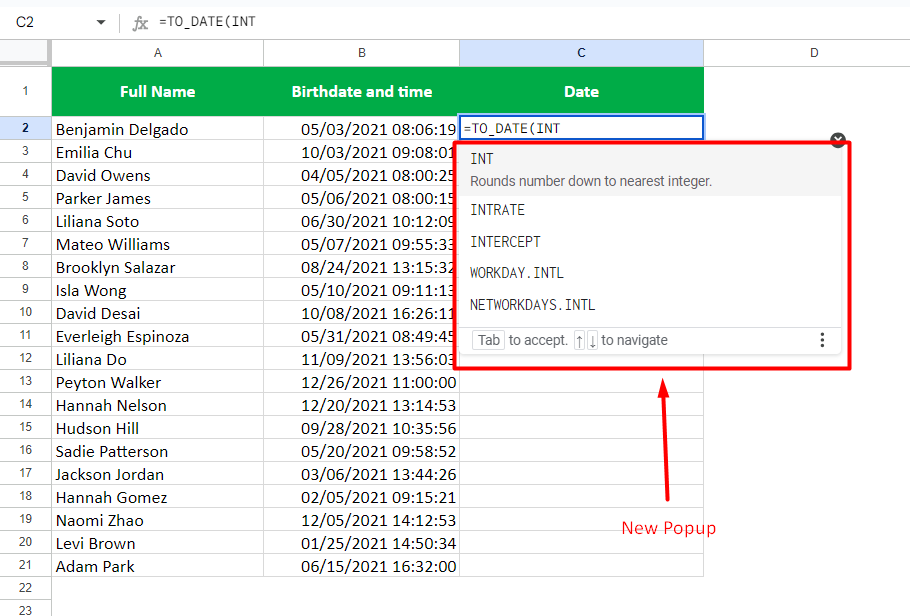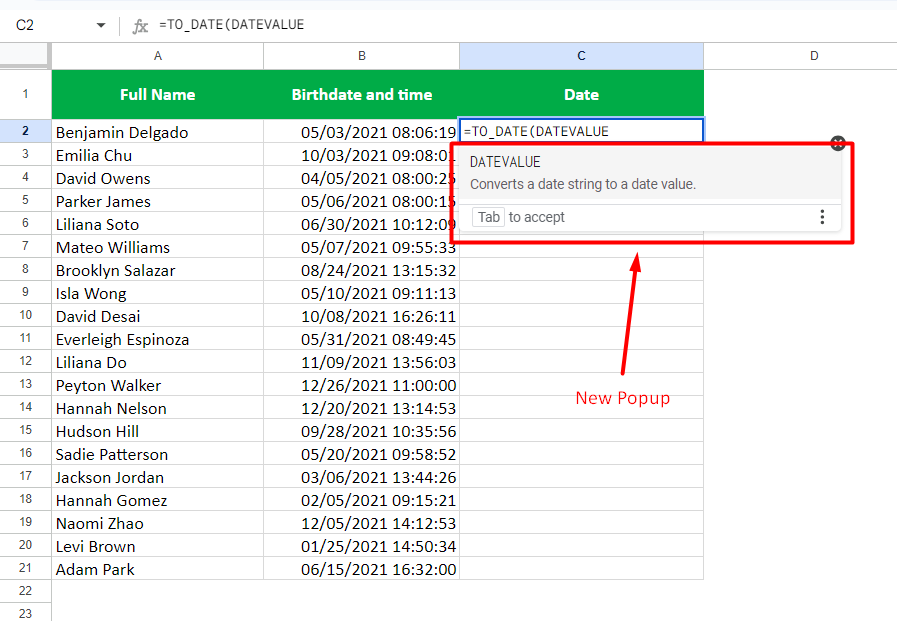# How to Convert Timestamp to Date in Google Sheets (in 3 Ways)

Timestamps represent the DateTime values in Google Sheets and can be separated into Date and Time. You can use the TO_DATE function in Google Sheets and either the INT or DATEVALUE function to convert timestamps to dates.

After exporting a dataset from an online source a few times, we noticed that the cell contains a combined date and time.

So, for further data analysis and organization, separating the date and time is pretty obvious.

Let us discuss all the possible ways to get a date from a timestamp. This article contains useful information about various functions and features of Google Sheets, so make sure to read it until the end.

Consider downloading the following file containing the dataset we will use to demonstrate getting the date from a timestamp.

It will surely improve your knowledge of various functions in Google Sheets.

## What Functions Are Used to Convert Timestamp to date in Google Sheets?

The TO_DATE function needs to be coupled with either the INT or DATEVALUE function to extract the date from a timestamp.

So, let’s understand these functions first.

### The TO_DATE function

It is a simple formula with the following syntax,

``=TO_DATE(value)``

Here, the “value” argument must be replaced with the number you wish to convert to a date. It accepts integer values only.

You may say timestamps are not the numbers! So, how would the function TO_DATE apply to them?

But note that the timestamp 3/22/2020 10:05:55 is stored as the number 43912.420775463 in Google Sheets.

In other words, timestamps are also numbers and are accepted by the TO_DATE function.

IMPORTANT: Consider the following example where we used the TO_DATE function on a timestamp. It returned the date as an output.

But if you change this cell’s format, you will know that it is date and time, but the time part is hidden.

So, the TO_DATE function will return the date from the timestamp. But, it won’t be just a date; instead, the date and time, but the time will be hidden.

### The INT function

This function rounds the given number to the nearest integer, which is less than or equal to it.

The general syntax for the INT function is as follows:

``=INT(value)``

The “value” argument must be replaced with the decimal number you wish to round to the nearest integer number. You can either manually type the decimal number or select the cell reference holding the decimal number.

### The DATEVALUE function

It is a simple function in Google Sheets that converts the date to a serial number.

The general syntax is as follows,

``=DATEVALUE(date_string)``

Here, the “date_string” argument must be replaced with the date you wish to convert to a serial number.

In the case of timestamps, the DATEVALUE function considers the date part of the timestamp and converts it to a serial number.

Now, let us get the date from the timestamp.

## Method #1 – Getting the date from the timestamp using the TO_DATE and INT function

Using the TO_DATE and INT Functions, below are the steps to convert timestamp to date in Google sheets.

• Click on the desired cell and type “`=to_date`
• Select the first option from the popup or pressTab” on your keyboard
• Now, for the “value” argument of the TO_DATE function, type “INT
• As the INT is a function in Google Sheets, again, you will see a popup• Choose the first option or press the “Tab” key
• Provide the cell reference as “B2
• Complete the brackets using “))” on your keyboard
(Note that there are two brackets to close; one is for the TO_DATE function, and the other is for the INT function. Hence, we have put two “)” to complete this formula)
• PressEnter” key

Our final formula will be as below,

``=TO_DATE(INT(B2))``

Where the B2 acts as the cell reference value holding the timestamp.

## Method #2: Getting the date from the timestamp using the TO_DATE and DATEVALUE function

Using the TO_DATE and DATEVALUE Functions, below are the steps to convert timestamp to date in Google sheets.

• Click on the desired cell and type “=to_date
• Select the first option from the popup or pressTab” on your keyboard
• Now, for the “value” argument of the TO_DATE function, type “DATEVALUE
• As the DATEVALUE is a function in Google Sheets, again, you will see a popup• Choose the first option or press the “Tab” key
• Provide the cell reference as “B2
• Complete the brackets using “))” on your keyboard
(Note that there are two brackets to close; one is for the TO_DATE function, and the other is for the DATEVALUE function. Hence, we have put two “)” to complete this formula)
• Press “Enter” key

Our final formula will look something like below,

``=TO_DATE(DATEVALUE(B2))``

Where the B2 is the cell reference value holding the timestamp.

## How to convert a timestamp to date using the SPLIT function in Google Sheets

The SPLIT function breaks down the timestamp into date and time. Refer to the following image.

The general syntax for this formula is as below,

``=SPLIT(text, delimiter, [split_by_each], [remove_empty_text])``

Here is a quick explanation for the first two arguments of the SPLIT function,

• text” – It needs to be replaced with the text that you wish to divide
• delimiter” – Here, you define the character[s] that will split the given text

The remaining two arguments are optional and beyond the scope of this article, so we will not discuss them.

For this function to work, you must prepare two separate columns, as shown in the image below. Also, make sure to keep all the cells from these new columns empty. Otherwise, the function will return an error.

Now, let us use the SPLIT function to convert a timestamp to a date in Google Sheets:

• Type “=split
• Select the first option from the popup or pressTab” on your keyboard
• Now, for the “text” argument, we provide the cell reference as “B2
• Press “,” on your keyboard to move to the next argument
• Next, as the date and time in the timestamp are separated by the space, we will put “ “ in the place of the “delimiter” argument
(Please put the space in double quotation marks; otherwise, the formula will not work.)
• Complete the brackets using “)
• PressEnter

The final formula should look like this:

``=SPLIT(B2," ")``

## Conclusion

That’s all about extracting a date from a timestamp in Google Sheets.

Also, note that if you just wish the timestamp to appear as a date visually, you can change its formatting by simply going to the “Format” tab of the main menu and then selecting the “Number” option followed by “Date”.

I hope this article taught you about everything when it comes to getting the date and time from the timestamp.

Feel free to comment below if you are still stuck or facing any particular issue. I will answer all your questions as soon as possible.